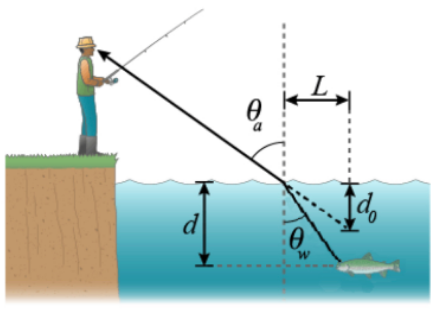# Problem:  A fisherman spots a fish underneath the water. It appears that the fish is d0 = 0.78 m under the water surface at an angle of θa = 68° with respect to the normal to the surface of the water. The index of refraction of water is nw = 1.3 and the index of refraction of air is na = 1. (a) The perpendicular distance from the apparent position of the fish to the normal of the water surface shown in the figure is L. Express L in terms of tan θa and d0. (b) Solve for the numerical value of L, in meters. (c) Express the sine of the angle θw, in terms of θa, nw, and na. (d) Solve for the numerical value of in degrees. (e) Now assume that the real position of the fish is directly below the apparent position, as shown in the figure. Express the real depth of the fish, d, in terms of L and tanθw.

###### FREE Expert Solution

Snell's law:

$\overline{){{\mathbf{\eta }}}_{{\mathbf{1}}}{\mathbf{s}}{\mathbf{i}}{\mathbf{n}}{{\mathbf{\theta }}}_{{\mathbf{1}}}{\mathbf{=}}{{\mathbf{\eta }}}_{{\mathbf{2}}}{\mathbf{s}}{\mathbf{i}}{\mathbf{n}}{{\mathbf{\theta }}}_{{\mathbf{2}}}}$

Part (a)

It's observable that tan θa = L/d0

Therefore, L = d0 tan θa

The expression for L is d0 tan θa

Part (b)

The numerical value of L is:###### Problem Details

A fisherman spots a fish underneath the water. It appears that the fish is d0 = 0.78 m under the water surface at an angle of θa = 68° with respect to the normal to the surface of the water. The index of refraction of water is nw = 1.3 and the index of refraction of air is na = 1.(a) The perpendicular distance from the apparent position of the fish to the normal of the water surface shown in the figure is L. Express L in terms of tan θa and d0.
(b) Solve for the numerical value of L, in meters.
(c) Express the sine of the angle θw, in terms of θa, nw, and na.
(d) Solve for the numerical value of in degrees.
(e) Now assume that the real position of the fish is directly below the apparent position, as shown in the figure. Express the real depth of the fish, d, in terms of L and tanθw.